Documentation

## Applications

Last updated on May 18, 2020 by Mark Fric

# Envelopes indicator

Page contents

in this example we’ll add Envelopes indicator to StrategyQuant X.

MQL code for this indicator can be found here: https://www.mql5.com/en/code/7975

This indicator is also already build-in into MetaTrader, you can call it from MQL code using iEnvelopes method. This is how the indicator looks on chart: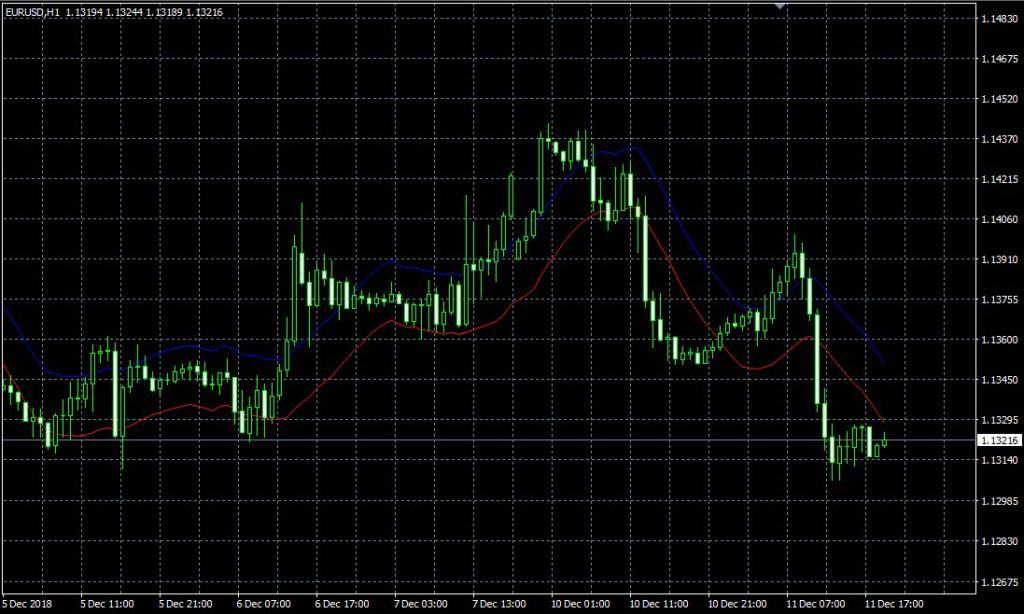There are few steps to follow to add new indicator to SQ X:

1. Adding new indicator building block
we’ll create a new indicator snippet and update its code to compute Envelopes indicator.
2. (Optional, recommended) Testing new indicator in SQ X vs data from MT
during or after implementation we should compare the indicator values computed by SQ with those computed by MQL – to make sure the indicator is implemented correctly
3. Adding translation of Indicator block into the language of target platform
indicator works in SQ now, but strategies using this indicator don’t. We have to add templates ot generate source code for this indicator for every trading platform we want to support

to do this, we’ll open Code Editor.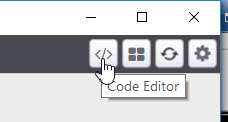There we click on Create new button in the toolbar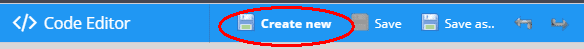and in the popup dialog we enter ‘Envelopes‘ as new indicator name and leave Indicator as the Snippet type.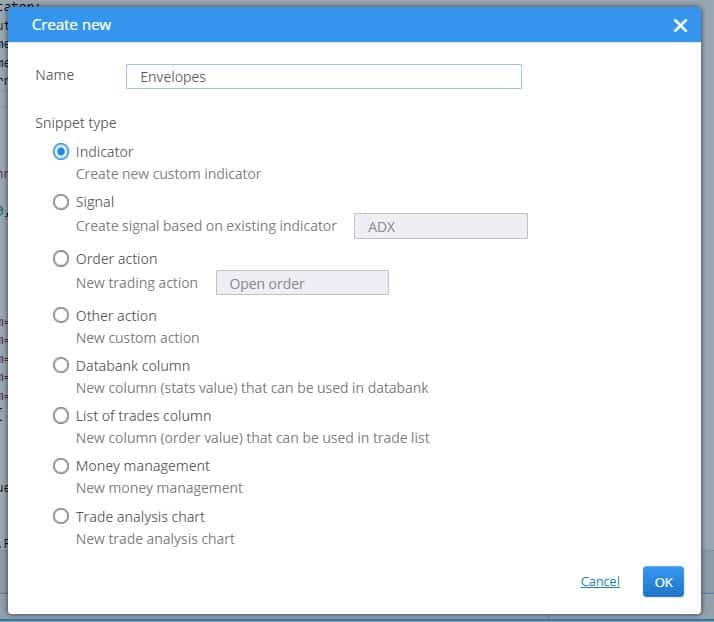Click OK, and new indicator will be created.

You can see it in Navigation tree, in Snippets -> SQ -> Blocks -> Indicators. It has its own folder named ‘Envelopes’, and indicator snippet code is in file Envelopes.java

The convention in StrategyQuant is that every indicator is in its own folder – it is because later we might want to create signals for this indicator, and all the related snippets will be in the same folder.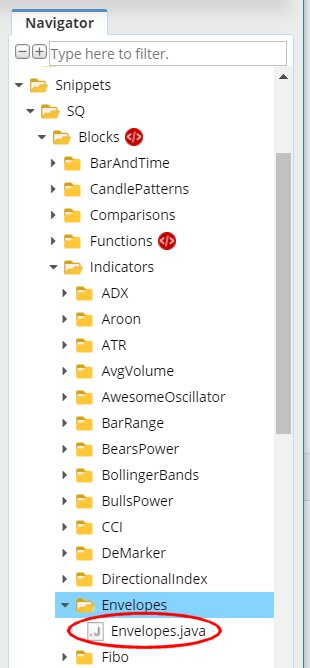This action created new file for our Envelopes indicator and opened it in editor. You can see that the indicator is a class and it already has some structure.

Every indicator is extended from IndicatorBlock class and it must implement one method:

• OnBarUpdate() – method where indicator value is computed and stored to one of the output buffers. It Is called for every bar on chart.

When you’ll check the source code you should notice few things.

First, snippets in StrategyQuant use annotations a lot – @Parameter, @Output, @BuildingBlock – these annotations are used to declare a special property of a given variable or class – that the variable is a public parameter or output value, or that class is an indicator block.

Secondly, once you create and compile an indicator, you can call it from other methods or indicators by using Indicators.YourIndicator(YourIndicatorParameters). This is how one indicator can be called from another – we’ll use it also in Envelopes to call Moving Average indicators, we’ll get to it later.

We’ll go through the source code of the default indicator class created from template step by step:

```package SQ.Blocks.Indicators.Envelopes;

import com.strategyquant.lib.*;
import com.strategyquant.datalib.*;

import SQ.Internal.IndicatorBlock;```

this is standard Java declaration of a package and imports of required classes – the ones we use in our own class.

```@BuildingBlock(name="(XXX) Envelopes", display="Envelopes(#Period#)[#Shift#]", returnType = ReturnTypes.Price)
@Help("Envelopes help text")
public class Envelopes extends IndicatorBlock {```

Annotated definition of our indicator class, telling the system that it is a building block with given name.

• name field is what is displayed in UI when choosing building blocks.
• display field is what is displayed In Wizard with parameters. You can control which parameters are shown and in what place
• returnType is a type of indicator, it says what kind of value this indicator computes. It is used so that StrategyQuant knows which types should be compared with what. For example, it wouldn’t compare CCI (which returns Number) with Bolinger Bands (which returns Price).

There are basically three return types an indicator can have:

• Price – indicator computes price and is displayed on price chart – like Bollinger Bands, Moving Average, etc.
• Number – indicator computes number that is displayed on its own chart – like CCI, RSI, MACD, etc.
• PriceRange – indicator computes price range (difference f two prices) – like ATR

Other return types are used in another types of building blocks.

In our case Envelopes is computed from moving average of a price, so it is displayed on the price chart. This means its return type is Price.

```@Parameter
public DataSeries Input;

@Parameter(defaultValue="10", isPeriod=true, minValue=2, maxValue=1000, step=1)
public int Period;

@Output
public DataSeries Value;```

what follows are indicator parameters. Every indicator can have multiple input parameters, the template creates just two of them as an example. Every parameter is annotated with @Parameter annotation, which could have several attributes.

The very first parameter is Input, it is the data series array from which the indicator is computed – it can be for example Open, High, Low or Close price.

Second parameter is Period, indicators usually have some period on which they are computed.

Third variable is Value, note that it has different annotation @Output. This means that this variable is not an indicator parameter but its output buffer. Indicators usually have just one output buffer, but they can have more – for example Bollinger band has Upper and Lower buffer.

There is one more hidden parameter Shift – it is by default in every indicator and it tells trading engine what value back it should look for. You generally don’t need to care about this parameter, it is used automatically.

Then there is a method:

`protected void OnBarUpdate() throws TradingException {...}`

This is the method where the indicator values are computed. It is called internally by SQ for every bar and it should compute indicator value for this bar and save it to the output buffer.

This is the source code of the standard indicator template. In the next step we’ll show the changes that must be made to implement our Envelopes indicator.

## Modifying the generated default template and implementing the indicator

The indicator created in the step 1 is a standard indicator template, it doesn’t yet compute Envelopes. To implement it we must do a few things:

### Update its @BuildingBlocks annotation

We’ll update the annotation of this indicator as follows:

```@BuildingBlock(name="(EP) Envelopes", display="Envelopes(#MA_Period#, #Deviation#)[#Shift#]", returnType = ReturnTypes.Price)
@Help("Envelopes indicator")
public class Envelopes extends IndicatorBlock {
```

This is the simplest part. We’ll just update name of the indicator and add the actual new parameters (see below) to the display attribute.

### Define its real parameters

The first thing to do is little tricky – we must change the type of the default Input parameter. In the standard template it is defined as follows:

```@Parameter
public DataSeries Input;```

it is parameter named Input, with type DataSeries. This is valid for a big portion of indicators that are computed from only one price. For example CCI, RSI, etc. indicators are usually computed from Close price. You can configure them to be computed from different price, for example from Open price, but still it is only one price array.

DataSeries type is an array of values type that holds values for Close prices, or for Open prices, or for Typical prices, etc.
However, if you’ll look at Envelopes MQL source code, you’ll see that it computes its values from one of the price values and from Volume.

To be able to access multiple price arrays at once we’ll use different type for output:

```@Parameter
public ChartData Input;```

ChartData
type is an object that represents the whole chart – you’ll have access to Open, High, Low, Close, Volume prices in the given chart. We need this so that we can cmpute the indicator according to the Applied price parameter.

Quick note – choosing the right type for input data variable  is not difficult:
If indicator is computed from one price  and there is no option to choose applied price, keep using DataSeries.
If it is computed from multiple prices or there is a choice – for example High, Low, Close, etc. – use ChartData.

Then there are indicator other parameters:

```@Parameter(defaultValue="14", isPeriod=true, minValue=2, maxValue=1000, step=1)
public int MA_Period;```

MA_Period is a “standard” period parameter for this indicator.

```@Parameter(defaultValue="0", minValue=0, maxValue=10, step=1)
public int MA_Moved;```

MA_Moved is shift of this indicator. In MQL code this parameter is named MA_Shift, but Shift is a reserved word in SQ, so we have to rename it.

```@Parameter(name="Method", defaultValue="0")
@Editor(type=Editors.Selection, values="Simple=0,Exponential=1,Smoothed=2,Linear weighted=3")
public int MA_Method;```

This parameter is moving average method. It is little bit more complex, because we define a selection list (combo box control) as edit control of this parameter. So when editing in Wizard, user will be able to choose from the predefined values.

```@Parameter(defaultValue="0")
@Editor(type=Editors.Selection, values="Close=0,Open=1,High=2,Low=3,Median=4,Typical=5,Weighted=6")
public int Applied_Price;```

The same applies to Applied_Price – this is a choice of inputs that can be used to compute Envelopes.

```@Parameter(defaultValue="0.1", minValue=0.01, maxValue=10, step=0.01, builderMinValue=0.05, builderMaxValue=1, builderStep=0.05)
public double Deviation;```

Deviation is the last input parameter of Envelopes indicator.

Please note that we also specified some values for min, max, and step for these parameters.

minValue/maxValue are the minimum/maximum ranges you’ll be able to set.

builderMinValue/builderMaxValue are optional ranges that builder in SQ will use when generating strategies – they can be smaller than maximum ranges defined in minValue/maxValue

defaultValue defines default value of this indicator.

step/builderStep defines step of the parameter value

### Define outputs

Envelopes indicator have two outputs – Upper and Lower – you can see two different lines in MT4 chart.

So we have to define two outputs as well:

```@Output(name="Upper", color=Colors.Green)
public DataSeries Upper;

@Output(name="Lower", color=Colors.Red)
public DataSeries Lower;```

The annotation @Output means that this is an output buffer for this indicator. Note that it has DataSeries type, which means it is an array of double values.

### Implement OnBarUpdate() method

If you’ll look at Envelopes MQL code you’ll see that it is quite simple, its MQL code is:

```  int start()
{
int limit;
if(Bars<=MA_Period) return(0);
ExtCountedBars=IndicatorCounted();
//---- check for possible errors
if (ExtCountedBars<0) return(-1);
//---- last counted bar will be recounted
if (ExtCountedBars>0) ExtCountedBars--;
limit=Bars-ExtCountedBars;
//---- EnvelopesM counted in the buffers
for(int i=0; i<limit; i++)
{
ExtMapBuffer1[i] = (1+Deviation/100)*iMA(NULL,0,MA_Period,0,MA_Method,Applied_Price,i);
ExtMapBuffer2[i] = (1-Deviation/100)*iMA(NULL,0,MA_Period,0,MA_Method,Applied_Price,i);
}
//---- done
return(0);
}```

If you’ll analyze it a little, you’ll see that the Upper and Lower values are computed using a simple formula:

Upper Band = [1+DEVIATION/100] * MA(PRICE, PERIOD)
Lower Band = [1-DEVIATION/100] * MA(PRICE, PERIOD)

where parameters DEVIATION, MA ,PRICE and PERIOD are configurable.

We can implement it in Java like this:

```    @Override
protected void OnBarUpdate() throws TradingException {
double ma = computeMA();
Upper.set( (1+Deviation/100d) * ma );
Lower.set( (1-Deviation/100d) * ma );
}```

Method OnBarUpdate() is called for every bar on the chart. Its responsibility is to compute value(s) of the indicator on this bar and store it to the output buffers.

So in our case we’ll compute both Upper and Lower values for the actual bar and store them into their buffers by calling Upper.set(), Lower.set().

Note that we use a special helper method computeMA() to compute moving average according to the MA_Method and Applied_Price parameters.

The code for this helper method is:

```private double computeMA() throws TradingException {
DataSeries MAInput;

switch(Applied_Price){
case 0: MAInput = Input.Close; break;
case 1: MAInput = Input.Open; break;
case 2: MAInput = Input.High; break;
case 3: MAInput = Input.Low; break;
case 4: MAInput = Input.Median; break;
case 5: MAInput = Input.Typical; break;
default: throw new TradingException(String.format("Undefined Applied price: %d !", Applied_Price));
}

switch(MA_Method){
case 0: return Indicators.SMA(MAInput, MA_Period).Value.get(MA_Moved);
case 1: return Indicators.EMA(MAInput, MA_Period).Value.get(MA_Moved);
case 2: return Indicators.SMMA(MAInput, MA_Period).Value.get(MA_Moved);
case 3: return Indicators.LWMA(MAInput, MA_Period).Value.get(MA_Moved);
default: throw new TradingException(String.format("Undefined MA Method: %d !", MA_Method));
}
}```

It is little bit longer, but understandable. First we’ll choose the correct price data according to Applied_Price parameter.

In the second step we’ll call the appropriate Moving average indicator on this input according to MA_Method parameter.

Let’s dissect one of the calls, for example: Indicators.SMA(MAInput, MA_Period).Value.get(MA_Moved):

• Indicators.SMA(MAInput, MA_Period) will call SMA indicator on MAInput input with MA_Period. SMA indicator has only one output buffer callled Value, so the computed indicator values will be stored there.
• we’ll get the buffer by simply calling .Value
• Value is a buffer (DataSeries type), same as our Upper and lower buffers, which means it is an array of double values, where each value belongs to one bar on the chart. To get value of the bar on Nth position, we should call Value.get(N).
In our case we’ll call .Value.get(MA_Moved), because we want value optionally shift some bars back, according to the parameter MA_Moved.

So the whole call Indicators.SMA(MAInput, MA_Period).Value.get(MA_Moved) will compute SMA with period MA_Period on the MAInput data, and returns its value MA_Moved bars ago.

Note that values in output buffer are indexed from zero, where zero is value of the most current bar.

So:

• Value.get(0) – returns value of the current bar
• Value.get(1) – returns value of the previous bar
• Value.get(2) – returns value of the bar before previous bar and so on

This is all, now when we hit Compile and then restart SQ we will see our new Envelopes indicator in Random Indicators Signals section.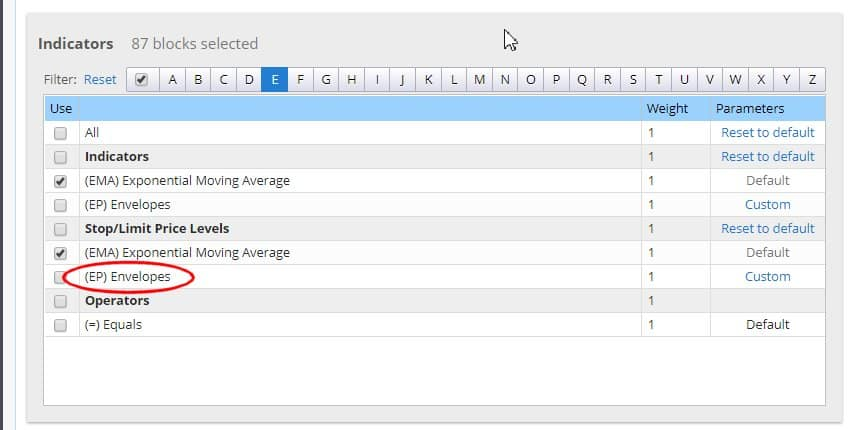```package SQ.Blocks.Indicators.Envelopes;

import com.strategyquant.lib.*;
import com.strategyquant.datalib.*;

import SQ.Internal.IndicatorBlock;

/**
* Indicator name as it will be displayed in UI, and its return type.
* Possible return types:
* ReturnTypes.Price - indicator is drawn on the price chart, like SMA, Bollinger Bands etc.
* ReturnTypes.Price - indicator is drawn on separate chart, like CCI, RSI, MACD
* ReturnTypes.PriceRange - indicator is price range, like ATR.
*/
@BuildingBlock(name="(EP) Envelopes", display="Envelopes(#MA_Period#, #Deviation#)[#Shift#]", returnType = ReturnTypes.Price)
@Help("Envelopes indicator")
public class Envelopes extends IndicatorBlock {

@Parameter
public ChartData Input;

@Parameter(defaultValue="14", isPeriod=true, minValue=2, maxValue=1000, step=1)
public int MA_Period;

@Parameter(defaultValue="0", minValue=0, maxValue=10, step=1)
public int MA_Moved;

@Parameter(name="Method", defaultValue="0")
@Editor(type=Editors.Selection, values="Simple=0,Exponential=1,Smoothed=2,Linear weighted=3")
public int MA_Method;

@Parameter(defaultValue="0")
@Editor(type=Editors.Selection, values="Close=0,Open=1,High=2,Low=3,Median=4,Typical=5,Weighted=6")
public int Applied_Price;

@Parameter(defaultValue="0.1", minValue=0.01, maxValue=10, step=0.01, builderMinValue=0.05, builderMaxValue=1, builderStep=0.05)
public double Deviation;

@Output(name="Upper", color=Colors.Green)
public DataSeries Upper;

@Output(name="Lower", color=Colors.Red)
public DataSeries Lower;

//------------------------------------------------------------------------
//------------------------------------------------------------------------
//------------------------------------------------------------------------

@Override
protected void OnBarUpdate() throws TradingException {
double ma = computeMA();

Upper.set( (1+Deviation/100d) * ma );
Lower.set( (1-Deviation/100d) * ma );
}

//------------------------------------------------------------------------

private double computeMA() throws TradingException {
DataSeries MAInput;

switch(Applied_Price){
case 0: MAInput = Input.Close; break;
case 1: MAInput = Input.Open; break;
case 2: MAInput = Input.High; break;
case 3: MAInput = Input.Low; break;
case 4: MAInput = Input.Median; break;
case 5: MAInput = Input.Typical; break;
default: throw new TradingException(String.format("Undefined Applied price: %d !", Applied_Price));
}

switch(MA_Method){
case 0: return Indicators.SMA(MAInput, MA_Period).Value.get(MA_Moved);
case 1:	return Indicators.EMA(MAInput, MA_Period).Value.get(MA_Moved);
case 2: return Indicators.SMMA(MAInput, MA_Period).Value.get(MA_Moved);
case 3: return Indicators.LWMA(MAInput, MA_Period).Value.get(MA_Moved);
default: throw new TradingException(String.format("Undefined MA Method: %d !", MA_Method));
}
}
}```

# Testing new indicator in SQ X vs data from MT

we just created (or we are in the process of develooping) our new indicator. How do we know that we implemented it correctly?

When you created your new indicator in StrategyQuant and it was successfully compiled, it should be also verified if it really works in the same way as in MetaTrader – in other words, if the values computed by it are the same as its values in MT4.

For this, we have an Indicators Tester tool in Code Editor. It works simply by comparing the values computed in SQ with values computed in MT4.

In general, it works in a few simple steps:

1. Use helper script to compute and export indicator data in MetaTrader
2. Copy the computed data files to proper location so SQ can find them
3. Configure and run indicator test in SQ

## Use helper script to compute and export indicator data in MetaTrader

As the first step we must prepare MT4 testing data – we must compute the indicator on a number of bars and save its computed values into a file.

For this we provide a simple EA that you can use – it is located in {SQ}/custom_indicators/MetaTrader4/Experts/SqIndicatorValuesExportEA.mq4

Add this EA to your MetaTrader, modify it to compute and output value of your indicator and run it in MT4 StrategyTester on any data – to make an optimal test it should run on at least 1000 bars.

Because Envelopes has two output buffers we have to run it two times – once for Upper, once for Lower buffer.

Here’s a modified code of this helper export script that computes Envelopes indicator:

```//+------------------------------------------------------------------+
//|                                   SQ_IndicatorValuesExportEA.mq4 |
//|                                                                  |
//|                    EA to export indicator values from MetaTrader |
//|                Output to: /{Data folder}/tester/files/******.csv |
//+------------------------------------------------------------------+

string currentTime = "";
string lastTime = "";

//+------------------------------------------------------------------+

int start() {
currentTime = TimeToStr(Time, TIME_DATE|TIME_MINUTES|TIME_SECONDS);
if(currentTime == lastTime) {
return(0);
}

double value;

// change the file name below
string fileName = "Envelopes_14_0_0_0_0.1_upper.csv";

int handle = FileOpen(fileName, FILE_READ | FILE_WRITE, ";");
if(handle>0) {
FileSeek(handle,0,SEEK_END);

// here is the indicator value
value = iEnvelopes(NULL, 0 , 14 , 0 , 0 , 0 , 0.1 , 1 , 1); // upper value
//value = iEnvelopes(NULL, 0 , 14 , 0 , 0 , 0 , 0.1 , 2 , 1); // lower value

FileWrite(handle, TimeToStr(Time, TIME_DATE|TIME_MINUTES|TIME_SECONDS), Open, High, Low, Close, Volume, value);
FileClose(handle);
}

lastTime = currentTime;
return(0);
}
```

it will compute the Envelopes inicator on MT4 by calling its internal method iEnvelopes() with proper parameters.

Please note that parameters can be configured – you don’t need to use default ones. It is a good practice to incude parameter values also in the name the output data file – as in our case “Envelopes_14_0_0_0_0.1_upper.csv”, so that we know it was generated with these parameters.

Now we can run this script two times in MT Tester: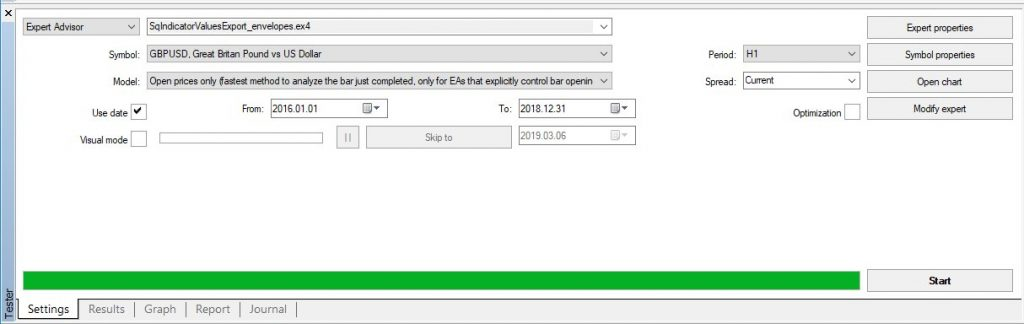When it finishes it should create data file with computed indicator values as well as Open, High, Low, Close prices for every bar. The file will be saved in MetaTrader4 -> {Data folder}/tester/files/Your_FILE_NAME.csv

We should see two files there:
Envelopes_14_0_0_0_0.1_upper.csv
Envelopes_14_0_0_0_0.1_lower.csv

## Copy the computed data files to proper location so SQ can find them

Copy these files to a folder

Create this folder if it doesn’t exists, SQ Indicaotrs Tester looks for files in this folder.

Now we have the data file prepared, let’s start a test in StrategyQuant.

## Configure and run indicator test in SQ

Co to Code Editor and click on Test indicators on the toolbar.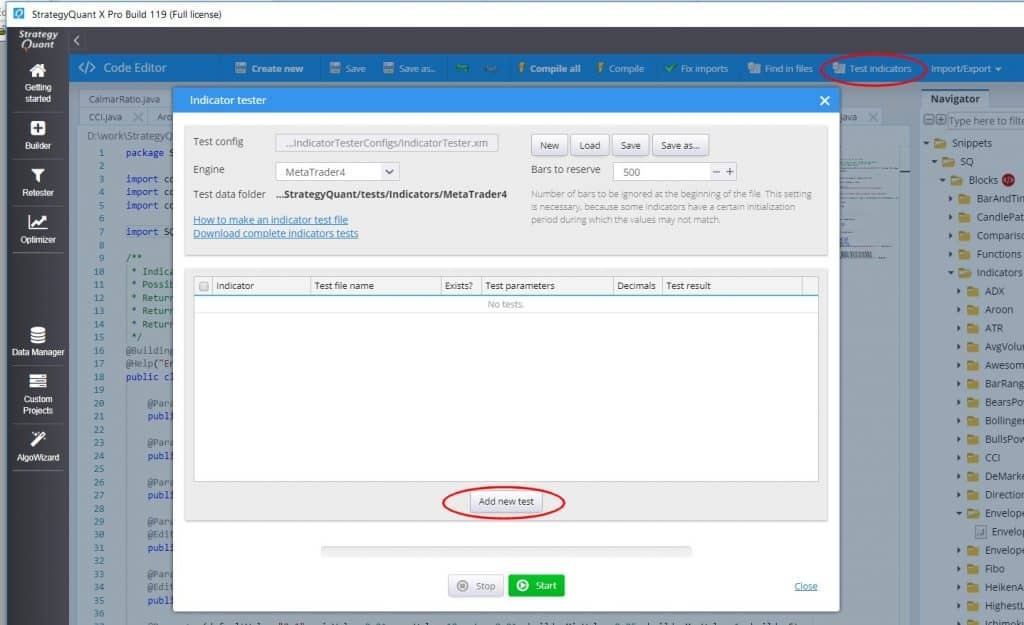It will open Indicator Tester dialog, click on Add new test. Add Envelopes indicators to the test, for both Upper and Lower output.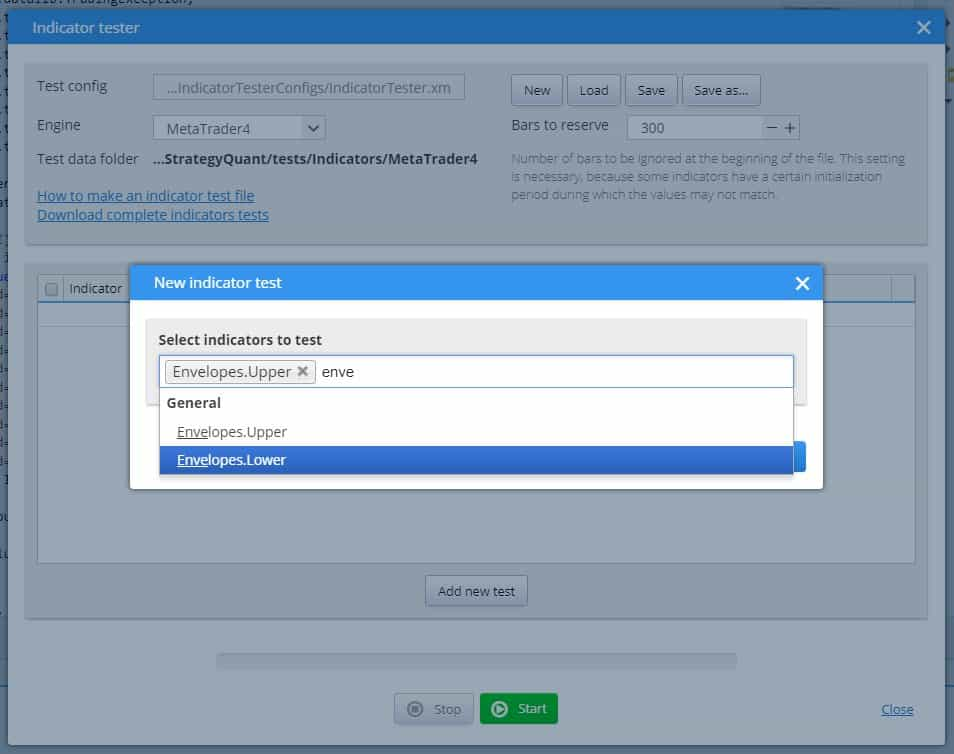You’ll see it in the table as follows. The last think we need to do is to modify the Test file names according to the actual test data file names created in previous step, and optionally also Test parameters, if you used other than default ones: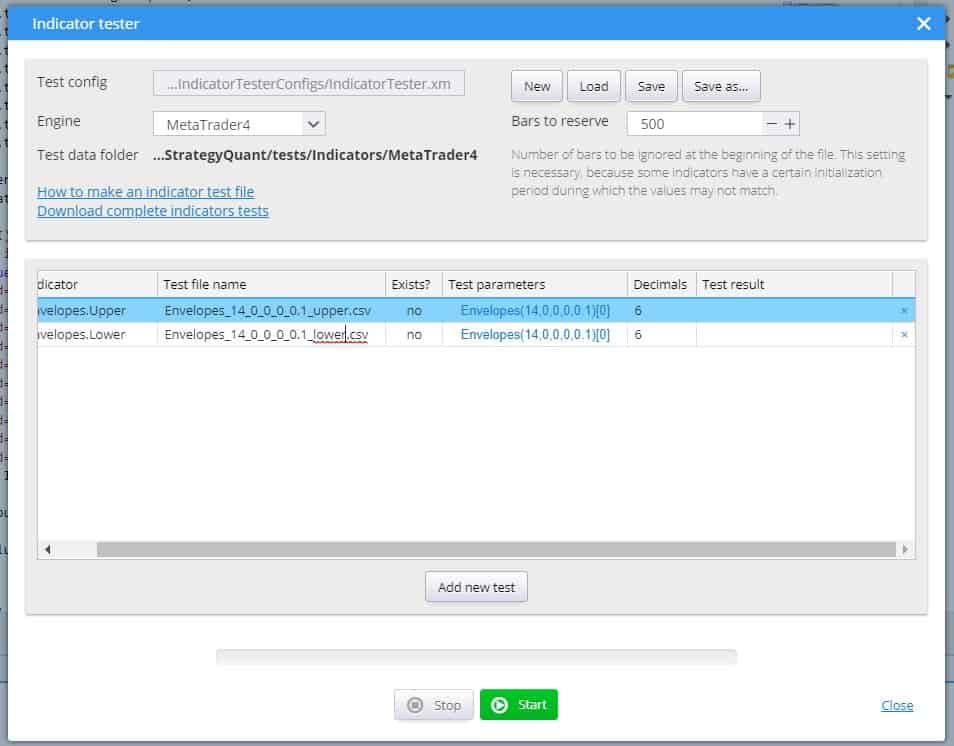When it is done click on Start button to run the tests.

In ideal case tests will be successful and values computed in SQ will match values computed in MT4: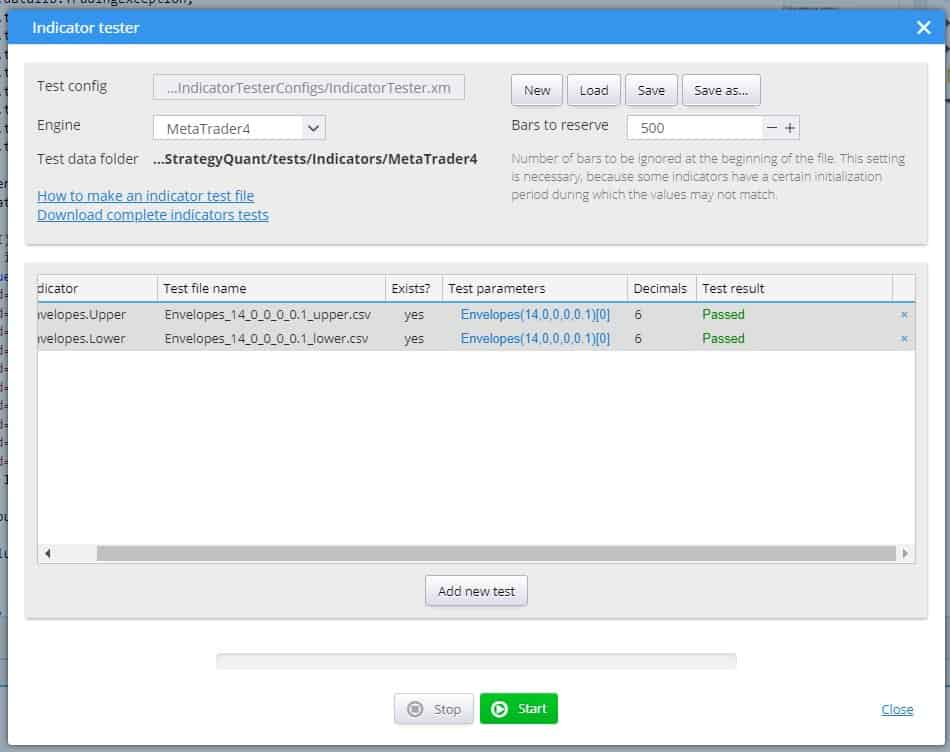If something fails there will be differences between values from SQ and MT4. You can click on the differences mesage to see them in the list: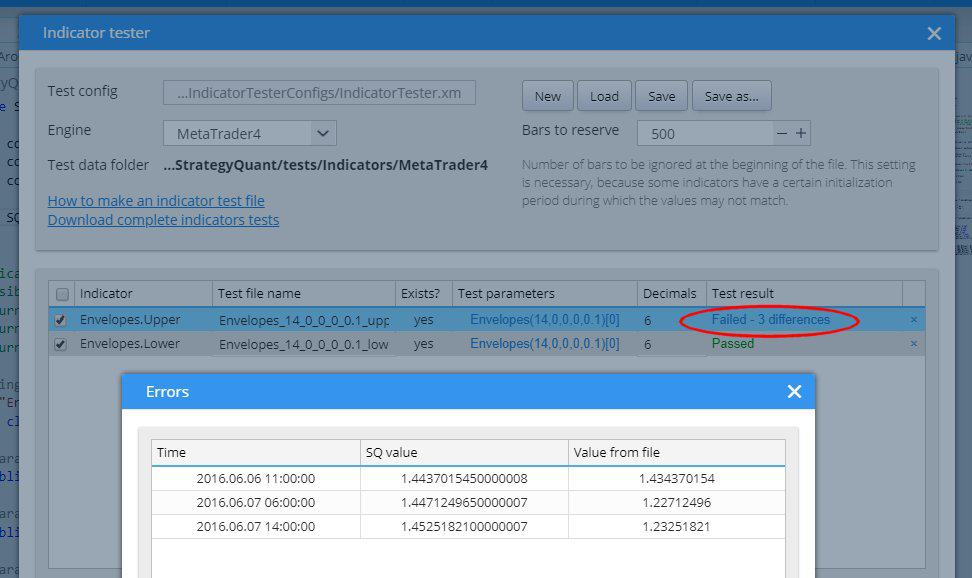If the test fails, first check if the test data generated in MT4 were using the same indicator parameters, there can be a mistake in that.

If the test data are correct, then there is something wrong with your SQ implementation of your indicator – it works differently than its MT4 counterpart and must be corrected.

# Adding translation of Indicator block into the language of target platform

Now the indicator is correctly working in StrategyQuant. You can use it to generate some strategies based on it, or use it in AlgoWizard. But we are not done yet.

If you’ll go to source code of your strategy, you’ll se an error message like this: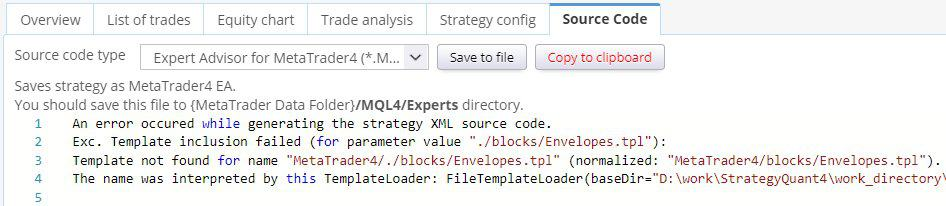It means that when generating Pseudo Code / MT4 / MT5 or Tradestation code, StrategyQuant couldn’t find a template that will translate the block from internal SQ XML format to the language of target platform.

So far we created a code for Envelopes to be computed inside StrategyQuant. But StrategyQuant doesn’t know how to translate this indicator into a code in your trading platform – it depends on platform itself.

In StrategyQuant, generated strategies are internally saved into XML format. When you’ll switch to Strategy XML and look for Envelopes indicator, you’ll see it is saved like this:

```<Item key="IsGreater" name="(&gt;) Is greater" display="#Left# &gt; #Right#" mI="Comparisons" returnType="boolean" categoryType="operators">
<Block key="#Left#">
<Item key="Envelopes" name="(EP) Envelopes" display="Envelopes(#Period#)[#Shift#]" help="Envelopes help text" mI="Envelopes" returnType="price" categoryType="indicator">
<Param key="#Chart#" name="Chart" type="data" controlType="dataVar" defaultValue="0">0</Param>
<Param key="#MA_Period#" name="MA _ Period" type="int" defaultValue="14" genMinValue="-1000003" genMaxValue="-1000004" paramType="period" controlType="jspinnerVar" minValue="2" maxValue="1000" step="1" builderStep="1">14</Param>
<Param key="#MA_Moved#" name="MA _ Moved" type="int" defaultValue="0" controlType="jspinnerVar" minValue="0" maxValue="10" step="1" builderStep="1">0</Param>
<Param key="#MA_Method#" name="Method" type="int" defaultValue="0" controlType="combo" values="Simple=0,Exponential=1,Smoothed=2,Linear weighted=3" builderStep="1">0</Param>
<Param key="#Applied_Price#" name="Applied _ Price" type="int" defaultValue="0" controlType="combo" values="Close=0,Open=1,High=2,Low=3,Median=4,Typical=5,Weighted=6" builderStep="1">0</Param>
<Param key="#Deviation#" name="Deviation" type="double" defaultValue="0.1" controlType="jspinnerVar" minValue="0.01" maxValue="10" step="0.01" builderMinValue="0.05" builderMaxValue="1" builderStep="0.05">0.1</Param>
<Param key="#Shift#" name="Shift" type="int" defaultValue="1" controlType="jspinnerVar" minValue="0" maxValue="1000" genMinValue="-1000001" genMaxValue="-1000002" paramType="shift" step="1" builderStep="1">1</Param>
<Param key="#Line#" name="Line" type="int" controlType="combo" values="Upper=0,Lower=1" defaultValue="0">0</Param>
</Item>
</Block>
<Block key="#Right#">
<Item key="Number" name="(NUM) Number" display="#Number#" help="Number constant" mI="Other" returnType="number" categoryType="other" notFirstValue="true">
<Param key="#Number#" name="Number" type="double" defaultValue="0" controlType="jspinner" minValue="-999999999" maxValue="999999999" step="1" builderStep="1">0</Param>
</Item>
</Block>
</Item>```

This is only a part of strategy XML that contains comparison of Envelopes with number. Note that it uses blocks (<Item>) IsGreater, Envelopes, Number.

SQ will look for templates to translate each of the XML blocks to the language of target platform. Templates for IsGreater and Number are by default in the system, but we are missing a template for Envelopes.

Templates are stored in Code subtree. There, each supported platform has its own folder, and inside it there is a subfolder /blocks that contains templates for every building block.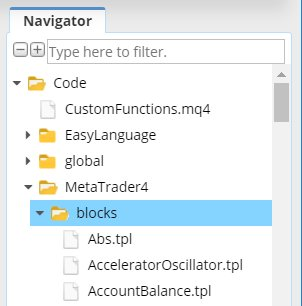Template files have .tpl extension and they are very simple. They use Freemarker template engine (https://freemarker.apache.org) to translate XML of the indicator into the target platform code.

Note that templates DON’T contain code to compute the indicator in the target platform, they contain code to CALL the indicator.

If you’ll check it you’ll see that there is no template Envelopes.tpl, hence source code shows a message that the template for it is missing.

## Adding Pseudo code template for a new block

When you’ll look at your Envelopessnippet in the Navigator window you’ll see that there is an error icon and when you mouse over it, you ‘ll see the error message – it is missing template code for every target platform.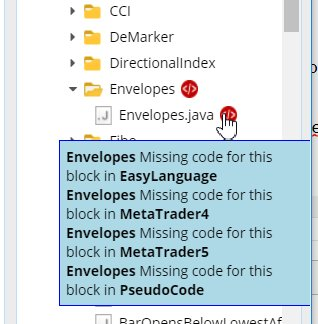The easiest way to add it is to click on Envelopes.java file with right mouse button to open pull down menu and there
choose action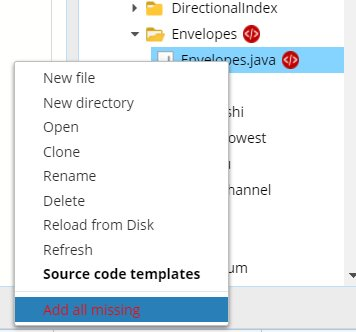This will add default templates for all target platforms.
When you’ll go to Code -> Pseudo code -> blocks you’ll see that it added template Envelopes.tpl with some default content.

You can see that template its quite simple, it is usually just one line.

Now that the template is there, you can again check the source code of your strategy.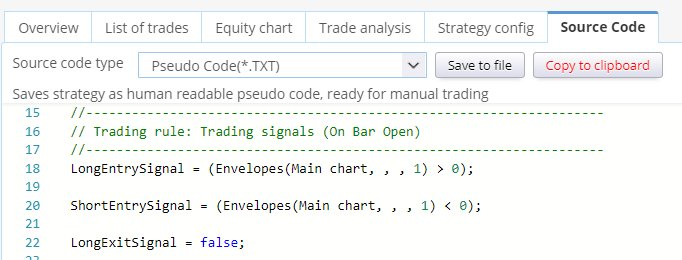You can see that the source code was produced, but it is not really correct.

It shows Envelopes(Main chart, , , 1) instead of real Envelopes parameters. It is because the Envelopes.tpl was created using default template, it didn’t use the real parameters of the indicators.

If you’ll check the PseudoCode/blocks/Envelopes.tpl code you’ll see it is as follows:

`Envelopes(<@printInput block true /> <@printParam block "#Param1#" />, <@printParam block "#Param2#" />, <@printShift block shift />)`

The methods printInput and printShift are default methods to print data input and shift values and they work correctly by default because every indicator has some chart/data input and shift.

But we don’t have parameters named Param1 and Param2 in our indicator.

Instead we have five other parameters there: MA_Period, MA_Moved, MA_Method, Applied_Price, Deviation.

So we’ll modify the template like this:

`Envelopes(<@printInput block true /> <@printParam block "#MA_Period#" />, <@printParam block "#MA_Moved#" />, <@printParam block "#MA_Method#" />, <@printParam block "#Applied_Price#" />, <@printParam block "#Deviation#" /><@printShift block shift />)`

Now if you’ll look at the Pseudo Code you’ll see it is displayed with correct parameter values: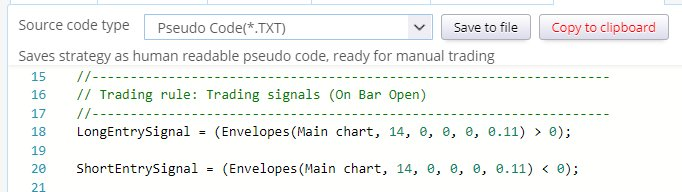As you can see, printing a parameter in a template is very simple – you just have to use method:

<@printParam block “#PARAMETER_NAME#” />

where PARAMETER_NAME is name of the parameter variable name from Java code.

There is still one thing that can be improved – parameters MA_Method and Applied_Price are displayed as numbers – we would like to display them as texts, so that we know what values were selected.

To do this, we can use method:

<@printParamOptions block “# PARAMETER_NAME #” “0=Option1,1=Option2,3=Option3” />

This method will translate the number value to the option based on its number.

Moreover, to be not overwhelmed wth unimportant information, we don’t need to display value of parameter MA_Moved in Pseud code at all. We can simply delete it from the template.

So our final Pseudo Code template code for Envelopes in PseudoCode will be as follows:

`Envelopes(<@printInput block true /> <@printParam block "#MA_Period#" />, <@printParamOptions block "#MA_Method#" "0=Simple,1=Exponential,2=Smoothed,3=Linear weighted" />, <@printParamOptions block "#Applied_Price#" "0=Close,1=Open,2=High,3=Low,4=Median,5=Typical,6=Weighted" />, <@printParam block "#Deviation#" /><@printShift block shift />)`

and it will produce output like this: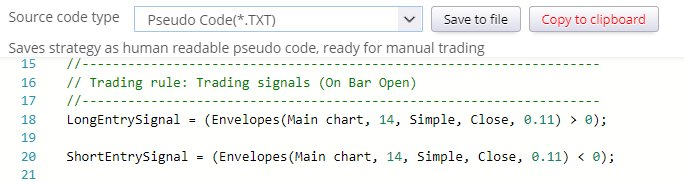In the previous step we added Pseudo Code template for our Envelopes indicator, so that we can see the strategy rules in Pseudo Code.

We must repeat this step for every target platform on which we want to use our strategy.

So let’s fix the template for MetaTrader MQL. There are two possibilities in MetaTrader:

• either the indicator is build-in into MetaTrader and then we can call it using its MQL function call,
• or it is a custom indicator and we must call it using iCustom MQL call.

We’ll show both ways, they differ only slightly in the code of the template.

### First possibility – indicator is build-in in MetaTrader

In this case you can find the indicator in the list of available indicators in the MT4 navigator, and you
can also find the method to call it in MQL Reference guide: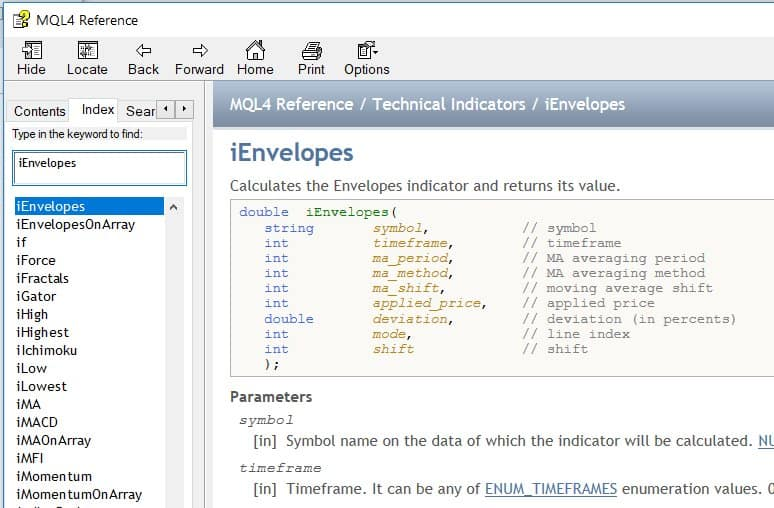From the MQL documentation we know that when calling this indicator we have to call function iEnvelopes() with the right parameters.

So we’ll open file Code / MetaTrader4 / blocks / Envelopes.tpl and change it as follows:

`iEnvelopes(<@printInput block />, <@printParam block "#MA_Period#" />, <@printParam block "#MA_Method#" />, <@printParam block "#MA_Moved#" />, <@printParam block "#Applied_Price#" />, <@printParam block "#Deviation#" />, <@printParam block "#Line#" />+1, <@printShift block shift />)`

What we did is that we renamed the method and added the correct parameters. Methods printInput and printShift produce correct output by default.

Note one more parameter – Line. Because Envelopes has two lines (Upper, Lower), also the call to iEnvelopes allows you to specify value for which line you want to retrieve – it is the 8th parameter mode, which is the line index.

This parameter in MQL is 1 for Upper, or 2 for Lower.

Line parameter is also by default created by SQ – just check the XML of this block. But it is zero based, and line index depends on order of @Output variables defined in the Java class. So in SQ 0 = Upper, 1=Lower. To get the same values as in MT4, we have to add 1 to the line value.

When we’ll go to MT4 source code we see it produced this output: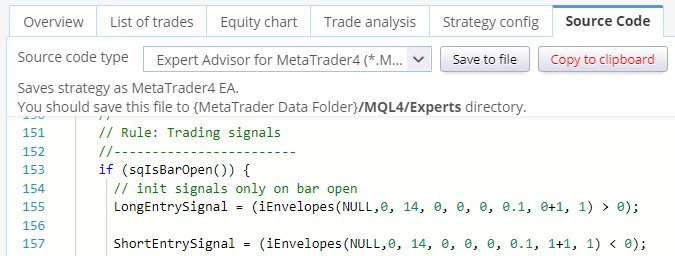Which is a correct way to call Envelopes indicator in MQL .

### Second possibility – it is custom (external) indicator for MetaTrader

Now what if the indicator is not build-in into Metatrader? The situation is only slightly more complicated.
As an example let’s say that Envelopes indicator doesn’t exists in MT4, and we have to use it as custom indicator.

and save it to a file  to {MT4} -> Data Folder/MQL/Indicators/Envelopes.mq4

This way it will become available in MetaTrader and can be used and called.

Custom indicators are called using MQL function iCustom: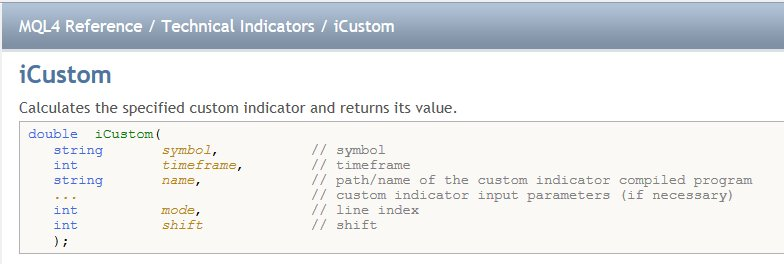So the correct call of Envelopes custom indicator in MQL would be:

`iCustom(Symbol, Timeframe, "Envelopes", MA_Period, MA_Moved, MA_Method, Applied_Price, Deviation, 1, Shift)`

to get the Upper line, and

`iCustom(Symbol, Timeframe, "Envelopes", MA_Period, MA_Moved, MA_Method, Applied_Price, Deviation, 2, Shift)`

to get the Lower line.

We define our template as follows:

`iCustom(<@printInput block />, "Envelopes", <@printParam block "#MA_Period#" />, <@printParam block "#MA_Moved#" />, <@printParam block "#MA_Method#" />, <@printParam block "#Applied_Price#" />, <@printParam block "#Deviation#" />, <@printParam block "#Line#" />+1, <@printShift block shift />)`

which again produces correct MQL code, using custom indicator call: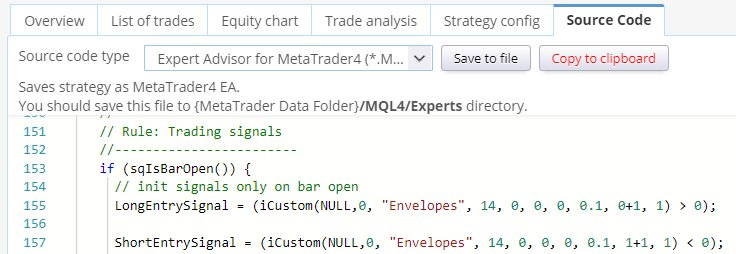The only difference from previous option is that we don’t use predefined MQL method iEnvelopes, but a general method iCustom that allows us to call any external custom indicator.